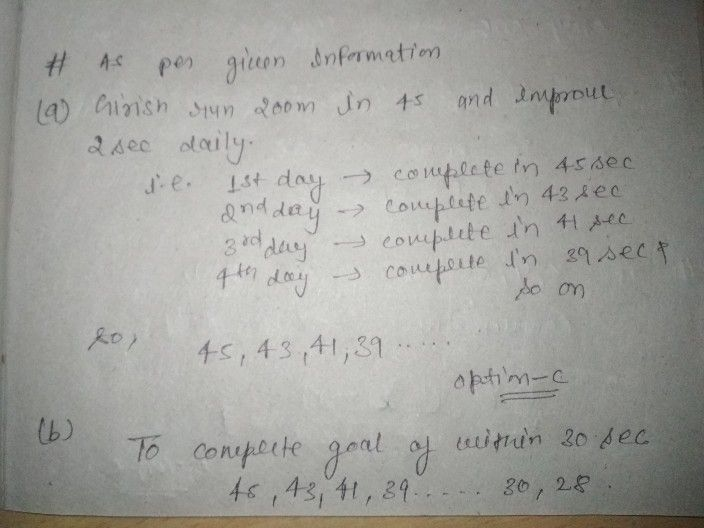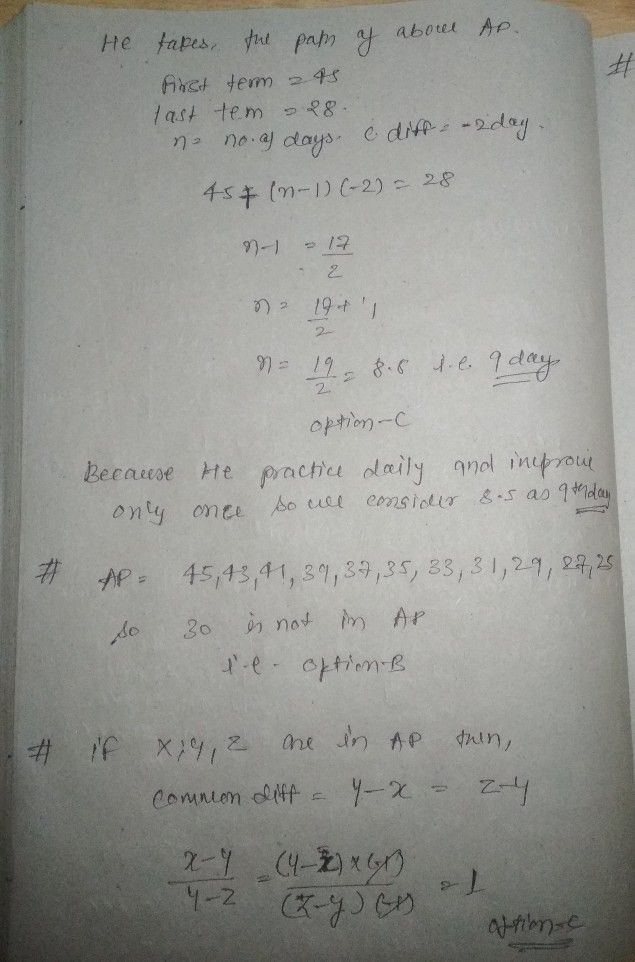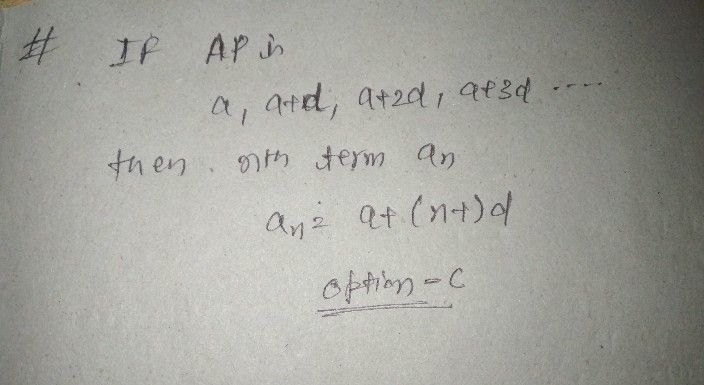Symbol
Problem17. SPRINT Sprint events in track and field usually consists of the $100m$ 200 m and 400 m race though 60 m dashes are also held on occasion. These races are largely based upon the athlete's ability to accelerate to his or her maximum speed in the quickest time possible. Girish wants to participate in the $200mS$ Sprint. He can currently run that distance $n45scC$ But he wants to do that in under $30scc$ With each day of practice, it takes him 2 sec less. Respond to the above situation by answering the questions given below. (a) Which of the following forms $anA.P$ for the above given situation. $\left(1\right)45,90,180,.....$ (i) $45,47,49$ . (iii) $45,43,41,.$ $...$ $\left(iy\right)$ $-45,-47,-48...$ .... (b) What is the minimum number of days he needs to practice till his goal is accomplished. (i) $8days$ $\left(1\right)8.5day3$ (iii) $9days$ (iii) none of these (c) Which of following term is not in the A.P of the above given situation. (i) 31 (ii) 30 (iii) 29 (iv) 27 (d) $1t\times .y.zacinA \,_{.}P_{,} lnc$ $\dfrac {x-y} {y-z}$ is equal to $\left(i\right)$ $→$ ½ (ii) 2 (iii) $1$ $\left(iy\right)$ $0$ (e) The nth term of the (i) $a+nd$ (ii) $nccaa+da+2d...B$ $\left(ii\right)$ $a+\left(n-1\right)d$ $a-\left(n-1\right)d$ (iv) $n+nd$
10th-13th grade
Algebra
Search count: 108
SolutionQanda teacher - NIMKIplease evaluate it if you wish give me reward that help me alot also rewarded coin will not deducted from your coin as that are on behalf of Qanda thankyou ☺️☺️Student
thank you mam